Finding the Median 1
Set 1 (10 Questions)
Students organize, represent, and interpret numerical and categorical data and clearly communicate their findings. Know the concepts of mean, median, and mode; compute and compare simple examples to show that they may differ.

From Mr. Anker Tests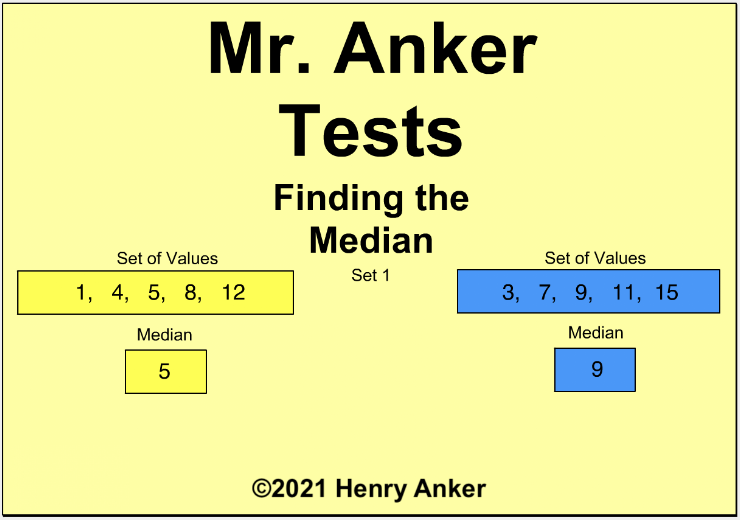Finding the Median Help Slide 1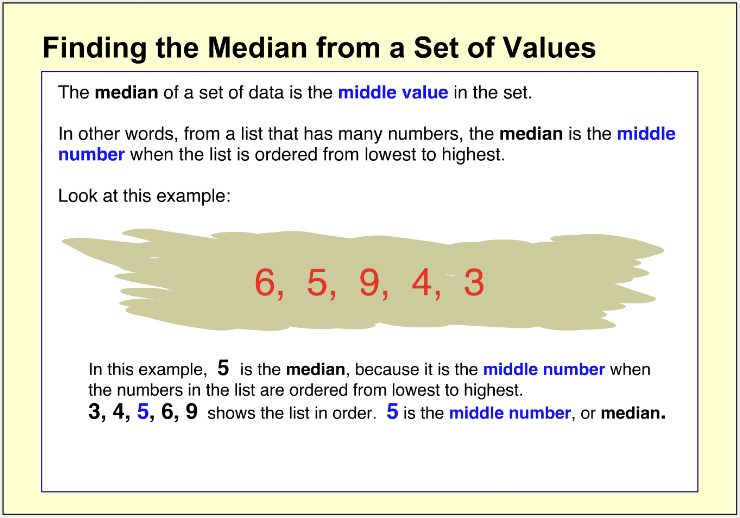Finding the Median Help Slide 2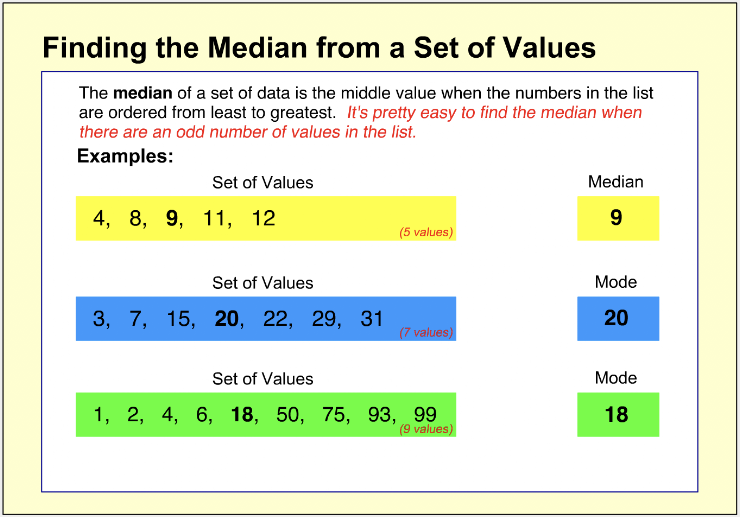Finding the Median Help Slide 3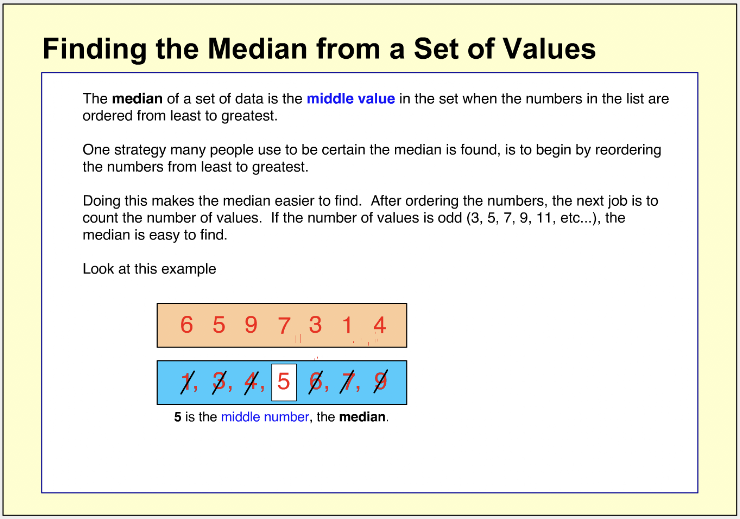Finding the Median Help Slide 4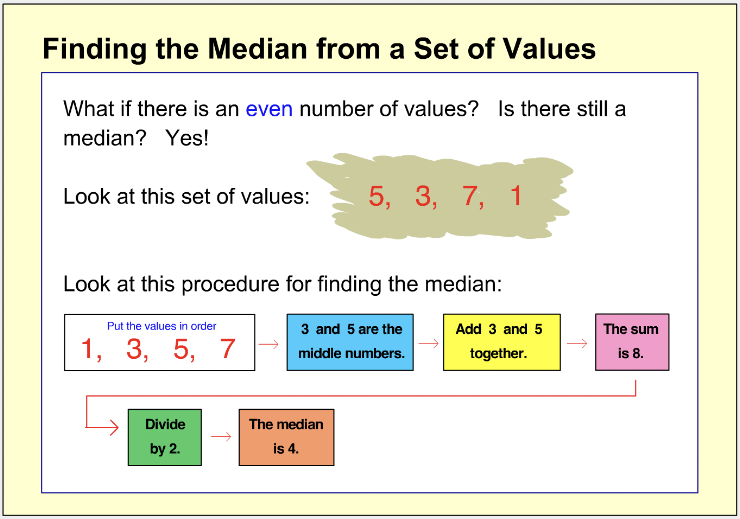Finding the Median Help Slide 5Finding the Median Help Slide 6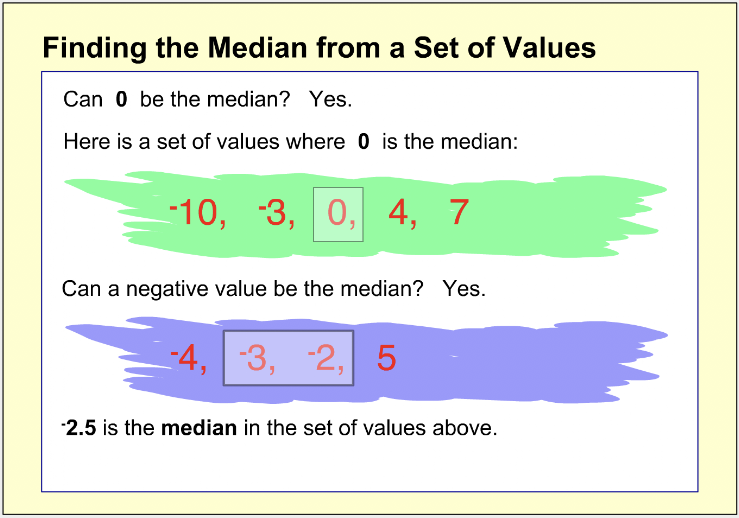1.  Which choice below is the median for the set of values shown? *
1 point2.  Which choice below is the median for the set of values shown? *
1 point3. Which choice below is the median for the set of values shown? *
1 point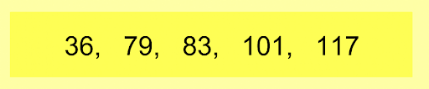4. Which choice below is the median for the set of values shown? *
1 point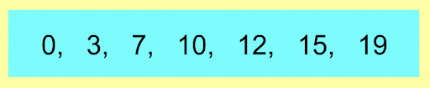5.  On the line below, enter the median for the set of values shown. *
1 point6.  On the line below, enter the median for the set of values shown. *
1 point7.  On the line below, enter the median for the set of values shown. *
1 point8.  On the line below, enter the median for the set of values shown. *
1 point9.  On the line below, enter the median for the set of values shown. *
1 point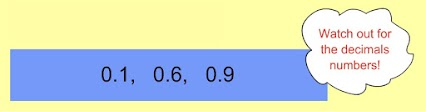10.  On the line below, enter the median for the set of values shown. *
1 point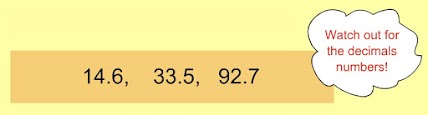Submit
Clear form